Internet of Things and Cloud Computing
Volume 3, Issue 3, October 2015, Pages: 42-47

Research on Innovating, Evaluating and Applying Multicast Routing Technique and Genetic Algorithm for Routing Messages in Service - Oriented Routing

Nguyen Thanh Long1, Nguyen Duc Thuy2, Pham Huy Hoang3

1Software Development Division III, Informatics Center of Hanoi Telecommunications, Hoan Kiem, Hanoi, VietNam

2Center for Applied Research and Technology Development, Research Institute of Posts and Telecommunications, NghiaTan, Cau Giay, Hanoi, VietNam

3Information Technology Institute, Ha Noi University of Science Technology, HaNoi, VietNam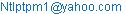(N. T. Long)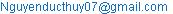(N. D. Thuy)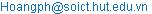(P. H. Hoang)

Nguyen Thanh Long, Nguyen Duc Thuy, Pham Huy Hoang. Research on Innovating, Evaluating and Applying Multicast Routing Technique and Genetic Algorithm for Routing Messages in Service - Oriented Routing. Internet of Things and Cloud Computing. Vol. 3, No. 3, 2015, pp. 42-47. doi: 10.11648/j.iotcc.s.2015030601.15

Abstract: MANET (short for Mobile Ad-Hoc Network) consists of a set of mobile network nodes, network configuration changes very fast. Each node will act as a router to maintain network operation. As some standard content based routing protocols, when a node publishes its content, it will broadcast data to network. The published content will be cached at all routers on the network. So when a node publish a request to get some content, it will prepare a subscription message then broadcast it. When any node on network received that subscription, it matches subscription’s content with published content that have been cached on it. If found then matched content will be transferred to requested node. Service Oriented Routing is inherited from the model of content based routing (CBR), combined with several advanced techniques such as Multicast, Genetic algorithm (GA) increase the data rate, and data encryption to ensure information security.

Keywords: MANET, QOS, Bandwidth, Time Slot, Service, GEN, GA

1. Introduction

Publish/Subscription Model is the interaction model is performed asynchronously in the content based routing .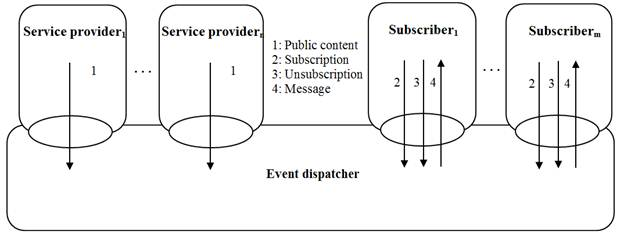Figure 1. Service based routing model.

Each network node must undertake the following roles: service provider, content receiver and router. In the article, we will make use of the advantages of these routing protocols and customize some important characteristics to construct novel routing protocol that is oriented service and guarantees quality of service.

Service oriented architecture allows flexible communication, the ability to provide location transparency for the transmission of information between applications.

Service-based network infrastructure is a new network interface in which the flow of messages is controlled by class of service that generated it.

2. Organization of Types of Messages

In order to perform service based routing, we have to organize data into messages, there are some message types:

1. Subcription / unsubcription message: to register/unregister node’s requested content. The message is structured with: the address of the subscriber and binding on the list of services and content requirements (constraints). The first constraint is service binding, followed by the content constraints. In particular, each constraint is a set of 3 components, has the form: (key,operator,value). In which key is a string, the operator is subject to value. i) If the value is the number then operators are: =, <, >, <=, >=, in; ii) If the value is a string then operators are: =, <, >, <=, >=, substring (sb), prefix (pf), postfix (ps).

2. Content message: to publish node’s content.

Content message structure: i) Source node address is the address of the node that generate message; ii) The next attribute1, attribute2,. .., attributen are the attributes of the message. The first attribute1 is the service information, the remaining attributes define the content of the message. Each attribute is a pair of name and value.

3. Advertisement message: to advertise node’s content, to direct subscriptions from subscribers to matched publishers, avoid to flood subscriptions all over networks. This message has structure similar to content message structure.

4. Update request (UR) and reply sender (RS) message pair: to update routing table. UR includes 3 fields: sender address and request number determine the uniqueness of UR. Timeout is the longest time the sender wait for an answer. The RS has three fields, two fields from the sender request, the field no.3 contains its all content based addresses. The RS spreads back the sender.

5. Route request (RREQ) and route reply (RREP) message pair: to detect routes. RREQ consists of 1) Source Address and 2) Request Number determine the uniqueness of RREQ; 3) Type is 1; 4) Timeout is time the sender waits for a response; 5) TTL is the maximum number of HOP of the route that message is passed on; 6) Route field records addresses of nodes; 7) Free TimeSlots records free timeslots of the nodes of the route; 8) Destination List field saves address list of destination nodes. When RREQ is received by a destination, it creates RREP to transmit to the source node. The RREP contains all fields from RREQ, besides the type field is set to 2 and doesn’t have destination list field.

3. Some Channel Access Techniques

A.  TDMA (Time Division Multiplex Access)

In TDMA, each subscriber is assigned some specific timeslots (TS) dynamically for sending data. After that it has to wait. A base station is responsible for coordinating the nodes. TDMA allows for low latency and guaranteed bandwidth.

B.  CSMA / CA (Carrier Sense Multiple Access / Collision Avoidance)

After waiting for a data transfer session ends, the node waiting a period of a random integer number of time units is specified. Node has waited completely first that will have right to transfer.

4. Multicast Routing

A.    Multicast model 

Assume a network is represented by a weighted graph G (T) = (V (T), L (T)), where V (T), L (T) denote the sets of nodes and links, respectively. Given a multicast tree T(s, M), where s is the source node of T: s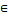V (T), M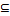V (T) \ {s} is the set of destinations. Define functions:

Delay (l): L (T) à R+, l is a link of this tree;

Cost (v): V (T) à R+, v is a vertex of this tree;

Bandwidth (l): B (l) à R+;

Delay(Ri) =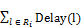(1)

Cost(Ri) =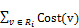(2)

Bandwidth (Ri) is defined in following section.

B.    Bandwidth calculation

Using timeslot  parameter to calculate bandwidth in MANET network using TDMA.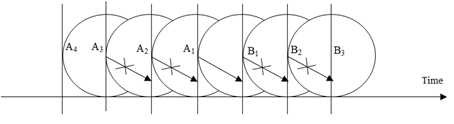Figure 2. Interference range and transmission range.

Status of each time slot is denoted by a (i, j), x (i, j) to represent time slot assignment’s result of time slot j of link i.

a(i, j) =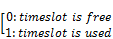(3)

x(i, j) =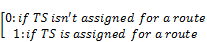(4)

Hence, in order for route has maximum bandwidth, have to assign maximum number of timeslots for each link. Several conditions that assignment has to satisfy: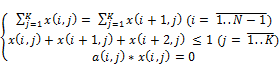(5)

1.   The calculation starts from the link that is in a state of having at least time slots. If there are more than one link that have equal minimum number of time slots, use the following formular to select link to start the algorithm:

a)  Define k constant numbers: W1> W2> …> Wk.

b)  TSh (h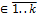), TSh is the free timeslots at time h.

c)   Check link independence by the formula:

INDEPENDENCE=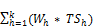(6)

d)  Choose the link that has INDEPENDENCE is maximum.

2.   Estimate bandwidth w of a route by formula:

w=min(t/3,g)                (7)

in which: t is the total of time slots, g is total of free time slots of start link.

3.   We call that link is Lmin (A, B). Select w time slots from free time slots of this link which satisfies Eq. 7.

4.   Choose w timeslots from T free timeslots of Lmin by following algorithm:

5.   Lmin has at most four neighbor links: li-2, li-1, li+1, li+2. Calculate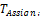=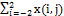, that is number of times the timeslot j is assigned for five links: li-2, li-1, li, li+1, li+2. Sort array T by ascending order, chose the first w timeslots.

6.   Delete selected w timeslots from free timeslots of li-2, li-1, li+1, li+2.

7.   If can’t choose w timeslots, reduce bandwidth estimation by 1, reassign bandwidth for this link.

8.   Choose w timeslots for each link from B to destination node or A to source node:

9.   For each link L(Bi, Bi+1), choose w timeslots from Ti free timeslots of this link by above algorithm in (4). If it cann’t be chosen then reduce the estimated route’s bandwidth by 1, reassign from the beginning. Remove this w timeslots for the next two links L(Bi+1, Bi+2) and L(Bi+2, Bi+3).

C.    Estimate latency

Based on analysis of some factors that affect the latency of transmission on the route (S, N1, N2, …, Nk, D). Estimate latency on each link by the formula:

D(l) =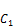* S +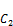* B +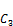* D +* M     (8)

Where: S is the size of data, B is bandwidth of the link l, D is the distance of the link, M is mobility of two nodes of the link.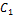,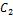,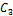,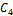are the weight of the factors S, B, D, M respectively.

D.    Algorithms for building minimum spanning tree

1. Build Shortest Path Tree based Multiple Paths (SPTM)

This method (A) builds multicast tree that has the shortest path by hop on the multipath route from the root node to the leaf nodes. Should include the concept of multipath routing, in every part of the route, find all possible paths. If there are multiple candidate paths to each path segment, they are sorted by shortest-path-first rule. The bandwidth of the route will equal to the total bandwidth of all possible paths: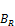=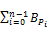, R={P0,P2, …,Pn-1}       (9)

2. Build Least Cost Tree based Multiple Paths (LCTM)

This method (B) builds multicast tree that has the total cost of all paths is minimum which is calculated by summing the cost of the links which form quality of service multicast tree. This kind of Multicast Tree can be built by PRIM algorithm. Hence the cost of the tree is equal to total cost of the links that have made tree: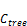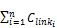(10)

On each part of route, find all possible paths based on the route detected messages pair as shown above.

3. Build Multiple Least Cost Trees (MLCT)

This method (C) builds multiple tree by using method (B). The algorithm: i) Build a multicast tree (T) by (B), if T is found the go to (ii), return the result; ii) scan this tree T, find any route R that doesn’t satisfy QOS. If not found, add T to the result set. Otherwise, remove R from route set (S). Go to (i). Dividing block of large data into some smaller blocks to send on multiple trees to the destination nodes. In destination nodes, combine some small packets into original data.

5. Find Optimal Route by GA

Some concepts of genetic algorithm

a)  Population: the set of routes (chromosome) detected by above algorithm in one time.

b)  Chromosome: each chromosome is consisted of identifiers (ID) of the nodes are on the route.

c)   Purpose: find the best route.

d)  Genetic operators: reproduction, mutation, crossover.

e)   Algorithm is specified in following steps:

1)  Make initial population from the detected routes.

2)  Next generations are generated by the evolution process: Evaluation by fitness function, Selection, Reproduction.

Until the number of generations reach a predefined threshold or the optimum solution is found.

A.  Establish the fitness function

Fitness function is established on the basic of some QOS parameters {. Define fitness of Ri by the function:

fi =(11)

Hop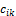between gene gk, gk+1.

B.  Establish Genetic Algorithm to find routes

The GA finds optimal QOS routes between a source (S) and a destination (D) by the following algorithm:

1.   Collect all possible routes between S and D.

2.   Assign weights for all parameters of these chromosomes.

3.   At each generation, choose the best chromosomes based on fitness function to get offsprings:

a.   Select two fittest routes based on fitness function;

b.   Execute crossover on R1 and R2 to get a new route: R3.

c.   Execute mutation on R3 to prune the low energy nodes.

Get the optimal QOS route R.

C.  Some techniques to execute crossover operations

1.   Two chromosomes must have at least a common gene.

2.   {R3} = R1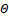R2,is crossover operation. Check if R3 has route loops. If true: i) remove this route and choose the next common gene; ii) remove the loops.

3.   If R3 is found, apply mutation for it. Finally add it to the next population.

D.  Some techniques to execute mutation operations 

1.         At first choose randomly the first sub-route of R3 = {s, N1, N2, …, Ni, …, d},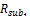= { s, N1, N2, …, Ni}. Delete randomly set of nodes after i.

2.         The remaining nodes:{Nj, Nj+1, …, d}, deg(j) is the set of neighbors of j;

3.         Ntemp = Nj; iloopcount = 0;

4.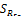= {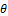};

5.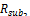= { Ntemp };

6.         deg(Ntemp) = {N1, N2, …, Nk}

7.         if |deg(Ntemp)| = 1 && i) if N1 <> d: exit; ii) if N1 = d: return= { Nj, N1 };

8.         if (|deg(Ntemp)| > 1) execute (9).

9.         if(iloopcount == 0) execute (10) else execute (15)

10.      For(i:=1; i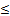k; i++){

11.      If((|deg(Ni)|==1)&&(deg(Ni)=={d})){

12.=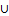{{ Ni, d }};}

13.      Else{

a.   If((i==1) && (iloopcount==0)){

i.        Ntemp = Ni;

ii.        iloopcount += iloopcount;

14.      Execute (6) for Ntemp}}

15.      For(i:=k; i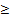1; i--){

16.      If((|deg(Ni)|>1) && (i==1))

17.      Return result;

18.      If((|deg(Ni)|==1)&&(deg(Ni)=={d})){

19.=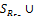{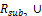{Ni,d };}}

6. Build Optimal Multicast Tree by GA

A.  Encoding multicast tree

Encode multicast trees into chromosomes. Use two arrays for each tree: (i) array S stores nodes’ ID in the pre-order of tree traversal depth first search algorithm; (ii) array T stores the parent’s ID of each node. This algorithm P is executed recursively:

a)  Assume R is root of the tree;

b)  Function is named Scan with two parameters: i) a node A that will be visited, ii) a reference parameter iCount, init iCount by 1, that stores the sequence number of current node A. So Scan is:

c)   Procedure Scan(byval R as Node, byref iCount as Integer)

d)  Visit R by storing R in its order in the array S:

1.   S[iCount] = R.ID;

2.   If iCount>1 then

i.        T[iCount] = R.parent.ID;

3.   End if

4.   Increase iCount by one: iCount += 1;

a)  Foreach(Node child in R.children)

b)  Execute Scan(child, ref iCount);

c)   End;

B.  Decoding chromosomes into multicast tree:

The pair of chromosomes S and T after applying GA will be decoded into multicast tree T by the algorithm:

a)  For k=2 to S.length Do

b)  L(T) = L(T)(S(k), S(T(k)));

c)   After the loop, get L(T) is the set of links of the tree T.

C.  Establish fitness function

F =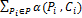, P is the set of chromosome’s parameters,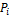,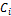are parameter and its weight for calculating the fitness level of each chromosome by function. For example, F = (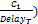*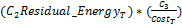. In each round, estimate each chromosome in the population by this fitness function to choose the two best chromosomes for applying genetic operators.

D.  Genetic operators

a)  Crossover operator: {Tnew1, Tnew2} = T1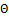T2, in which T1 = {T1first, A, T1second}, T2 = {T2first, A, T2second}, A is the common node of two chromosomes. So two result chromosomes can be:

b)  Tnew1 = {T1first, A, T2second}, Tnew2 = {T2first, A, T1second},

c)   Check Tnew1 and Tnew2 for eliminating any route cycle and having the same destination list.

d)  If they don’t satisfy these conditions then find the next common A of two chromosomes and execute (b).

e)   Loop until find two satisfied parent chromosomes (P) and get two child chromosomes or scan all common gene of P.

E.  Mutation operator

To eliminate low residual energy nodes of chromosome.

F.   Evaluation algorithm complexity

The algorithm has complexity O(n*(m+log(n))): m is the number of chromosomes and n is average number of genes of each chromosome.

7. Simulation and Evaluation

Simulate the SPTM:

Simulate with number of nodes from 50 to 1000 nodes, number of destination nodes is 10 and a fixed source node.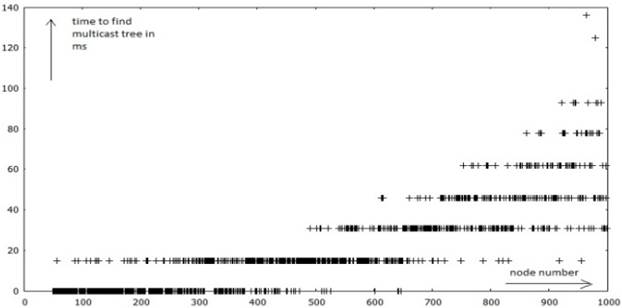Figure 3. SPTM Algorithm simulation diagram.

A.  Process to find optimal route by GA algorithm

a) Population size: this is about 20 to 30 chromosomes;

b) Crossover probability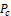0.2 to 0.9, mutation probability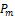0.05 to 0.2.

c) Chromosome size: this is about 20;

GA Simulation in 1000 times to find the optimal route.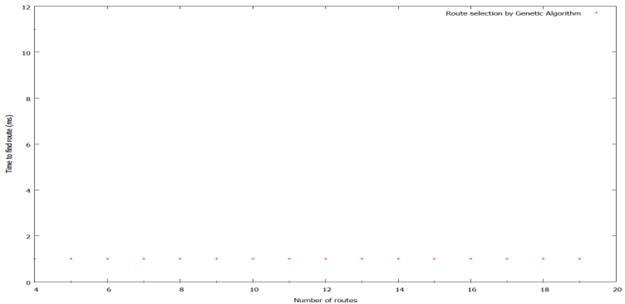Figure 4. Diagram for results of GA to find optimal route.

Time required to find the optimal route doesn’t increase when number of routes increase.

B.  Simulate to find optimal multicast tree by GA

a) Population size: this is about 2 to 100 chromosomes;

b) Crossover probability0.2 to 0.9, mutation probabilityis about from 0.05 to 0.2.

c) Chromosome size: about 20;

The number of simulation is 100 times: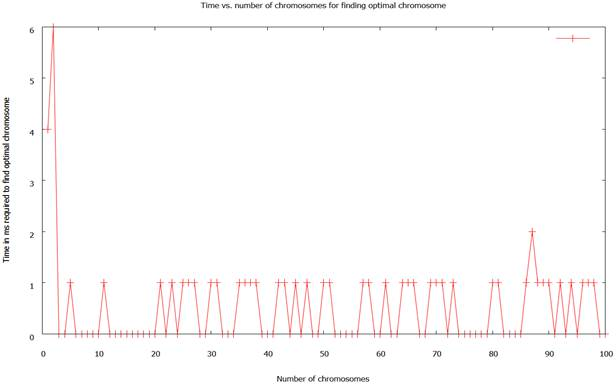Figure 5. Simulation GA to find optimal multicast tree.

The time required for algorithm execution is sometimes decreased when the number of multicast trees is increased. In general, this time is very stable.

8. Conclusion

We can improve the routing process in content based routing by using Multicast Tree. Use one of three strategies presented above to build trees with guaranteed bandwidth. The complexity of this algorithm is depended on length of the route LR and estimated route’s bandwidth W, that is O(LR* W). On the other hand, we can apply GA algorithms to find optimal route or multicast tree from the set of routes or multicast trees respectively. This process is carried out on the same time with the process to detect routes. So when the process to detect routes finishes, a little time later the GA process also finishes.

References

1. Fengyun Cao, Jaswinder Pal Singh. "Efficient Event Routing in Content-based Publish-Subscribe Service Networks" Infocom 2004.
2. R. Kalaiarasi, University Chennai, India. "PERFORMANCE ANALYSIS OF CONTENTION WINDOW CHEATING MISBEHAVIORS IN MOBILE AD HOC NETWORKS".
3. Antonio Carzaniga and Alexander Wolf. "Forwarding in a Content-Based Network," In Proc. SIGCOMM 2003.
4. Antonio Carzaniga, University of Colorado Boulder, Colorado 80309-0430 USA "A Routing Scheme for Content-Based Networking.
5. Jianping Li, the University of Tokyo, Japan, "Time Slot Assignment for Maximum Bandwidth in a Mobile Ad Hoc Network".
6. Huayi Wu, City University of Hong Kong, Hong Kong, "QoS multicast routing by using multiple paths / trees in wireless ad hoc networks".
7. Aisha-Hassan, Computer Engineering, IIUM, Malaysia, "Review of Multicast QoS Routing Protocols for Mobile Ad Hoc Networks".
8. J. Abdullah, Universiti Tun Hussein Onn Malaysia, Johor, Malaysia, "Effect of Maximum Node Velocity on GA-Based QOS Routing Protocol (QOSRGA) for Mobile Ad Hoc Network".
9. Nguyen Thanh Long, Nguyen Duc Thuy, Pham Huy Hoang, "Research on Innovating, Evaluating and Applying Multicast Routing Technique for Routing messages in Service-oriented Routing", Springer, ISBN: 978-1-936968-65-7, Volume Number 109, 2012.
10. K.Sasikala, V. Rajamani "A Modified Fuzzy Logic Routing for Wireless Mesh Network", International Journal of Computer Applications (0975 – 8887) Volume 60– No.2, December 2012.
11. Anjum A. Mohammed, Information Technology Department, College of Computer and Information Sciences,King Saud University, KSA, "Optimal Routing In Ad-Hoc Network Using Genetic Algorithm".

 Contents 1. 2. 3. 4. 5. 6. 7. 8.
Article ToolsAbstractPDF(384K)